# Additional Question Answers: Number System Notes | Study Mathematics (Maths) Class 9 - Class 9

## Class 9: Additional Question Answers: Number System Notes | Study Mathematics (Maths) Class 9 - Class 9

The document Additional Question Answers: Number System Notes | Study Mathematics (Maths) Class 9 - Class 9 is a part of the Class 9 Course Mathematics (Maths) Class 9.
All you need of Class 9 at this link: Class 9

Q1. Write four rational numbers equivalent to 5/7.

We have,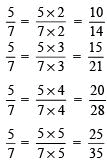Four rational numbers equivalent to 5/7 are 10/14, 15/21, 20/28, 25/35.

Q2. Find nine rational numbers between 0.1 and 0.2.

Let x = 0.1, y = 0.2 and n = 9.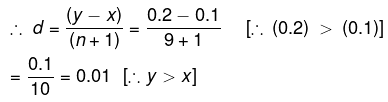The nine rational numbers between x and y are:
(x + d), (x + 2d), (x + 3d), (x + 4d), (x + 5d), (x + 6d), (x + 7d), (x + 8d) and (x + 9d).
Nine rational numbers between 0.1 and 0.2 are:
(0.1 + 0.01); (0.1 + 0.02); (0.1 + 0.03); (0.1 + 0.04); (0.1 + 0.05); (0.1 + 0.06); (0.1 + 0.07); (0.1 + 0.08) and (0.1 + 0.09)
The nine rational numbers between 0.1 and 0.2 are 0.11, 0.12, 0.13, 0.14, 0.15, 0.16, 0.17, 0.18 and 0.19.

Q3. Represent √2 on the number line (or on the real line).

• Draw XOX' and mark O as 0.
• Take OA = 1 unit.
• Draw AB ⊥ OX and cut off AB = 1 unit.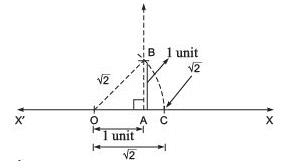• Now, OAB is a right triangle.
∵ OA2 + AB2 = OB2
⇒ 12 + 12 = OB2
⇒ 2 = OB2
⇒ OB =√2
• With centre O and OB as radius, draw an arc intersecting OX at C.
• Thus, OC = √2 on the real line XOX'.

Q4. Represent √3 on the real line.

• Draw XOX ' and mark O as 0 on it.
• Take OA = 1 unit.
• Draw AB ⊥ OX and cut off AB = 1 unit.
• Join OB such that OAB is a right triangle.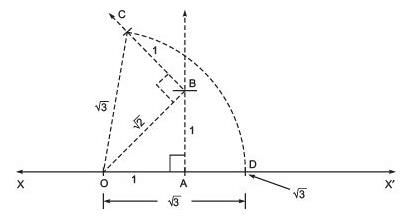∵ OA2 + AB2 = OB2
⇒ 12 + 12 = OB2
⇒ OB =√2
• Again, draw BC ⊥ OB and cut off BC = 1 unit. Join OC.
• Now, OBC is a right triangle.
∵ OB2 + BC2 = OC2
⇒ √22 + 12 = OC2
⇒ 2 + 1 = OB2
⇒  OC =√3
• With centre as O and radius as OC draw an arc to intersect OX at D.
• Thus, OD =√3 on the real line XOX'.

Q5. Express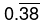as a rational number in simplest form.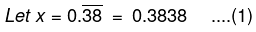⇒ 100x = 100 x (0.3838…)
⇒ 100x = 38.3838...(2)
Subtracting (1) from (2),
We have, 100x – x = (38.3838…) – (0.3838…)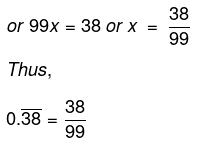Q6. Express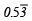in the form of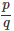.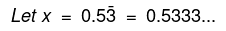∴ 10x = 10 x (0.5353…)
or 10x = 5.333... ...(1)
Also, 100x = 53.333... ...(2)
Subtracting (1) from (2),
⇒ 100x – 10x = (53.333…) – (5.333…)
⇒ 90x = 48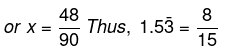Q7. Express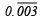is the form of p/q in the simplest form.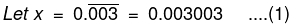∴ 1000x = 1000 x (0.003003…)
or 1000x = 3.003003... …(2)
Subtracting (1) from (2),
We have 1000x – x = (3.003003…) – (0.003003…)
⇒ 999x = 3
⇒ x = 3/999 = 1/333
Thus,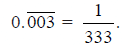Q8. Find the sum of  (3√3 +7√2) and (√3 - 5√2)

We have  (3√3 +7√2) + (√3 - 5√2)
⇒ √3 3+7√2 + √3 - 5√2
⇒ (3√3+√3) + 7√2 - 5√2)
⇒ √3(3+1) + √2(7-2)
⇒ √3(4) + √2(5) = (4√3 + 2√2)

Q9. Divide 15 √12 by 3√3.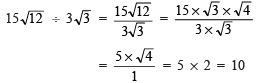Q10. Rationalize the denominator of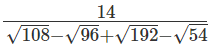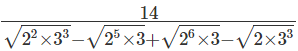(Prime factorize the numbers under the root in the denominator)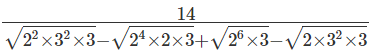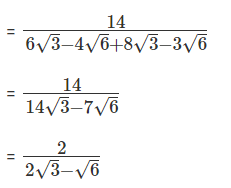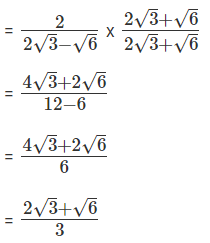Q11. Rationalize the denominator of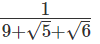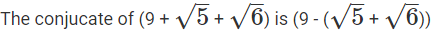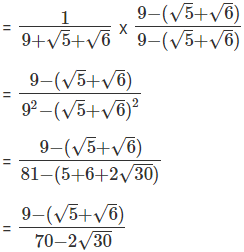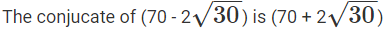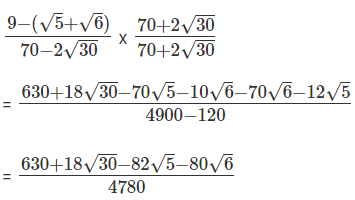Q12. If ‘a’ and ‘b’ are rational numbers and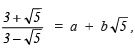find the values of ‘a’ and ‘b’.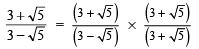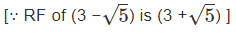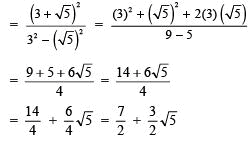Comparing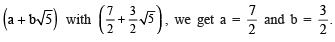Q13. If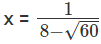, what is the value of x3 - 5x2 + 8x - 4?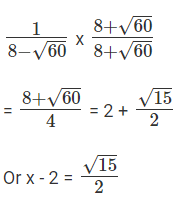(x - 2)3 = x3 - 6x2 + 12x - 8 ...(1)
(x - 2)2 = x2 - 4x + 4 ... (2)
(1) + (2) = x3 - 5x2 + 8x - 4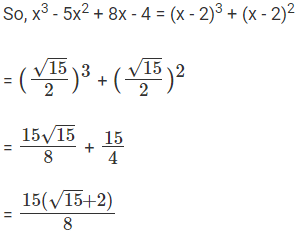Q14. Find the value of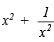when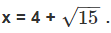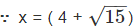∵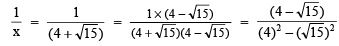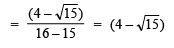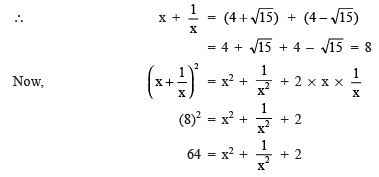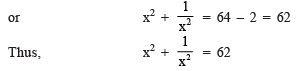Q15. Rationalise the denominator of 1/[7+3√3].

1/(7 + 3√3)

By rationalizing the denominator,

= [1/(7 + 3√3)] [(7 – 3√3)/(7 – 3√3)]

= (7 – 3√3)/[(7)2 – (3√3)2]

= (7 – 3√3)/(49 – 27)

= (7 – 3√3)/22

The document Additional Question Answers: Number System Notes | Study Mathematics (Maths) Class 9 - Class 9 is a part of the Class 9 Course Mathematics (Maths) Class 9.
All you need of Class 9 at this link: Class 9Use Code STAYHOME200 and get INR 200 additional OFF

## Mathematics (Maths) Class 9

73 videos|351 docs|110 tests

Track your progress, build streaks, highlight & save important lessons and more!

,

,

,

,

,

,

,

,

,

,

,

,

,

,

,

,

,

,

,

,

,

;